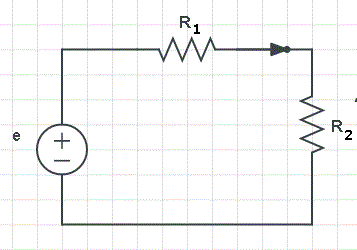# Ohm's Law with Examples

## Ohm's Law

Ohm's law states that the voltage V across a conductor of resistance R is proportional to the current I passing through the resistor (see circuit below). The relationship is written as.

V = R IWhich can also be written as
I = V / R and R = V / I.
The units are in Volts (V) for the voltage V, Amperes (A) for the current I and Ohms (Ω) for the resistance of R.

## Use Ohm's Law to Solve Simple Circuits Problems

Example 1
Find the current I through a resistor of resistance R = 2 Ω if the voltage across the resistor is 6 V.
Solution to Example 1
Substitute R by 2 and V by 6 in Ohm's law V = R I.
6 = 2 I
Solve for I
I = 6 / 2 = 3 A

Example 2
In the circuit below resistors R1 and R2 are in series and have resistances of 5 Ω and 10 Ω, respectively. The voltage across resistor R1 is equal to 4 V. Find the current passing through resistor R2 and the voltage across the same resistor.Solution to Example 2
We use Ohm's law V = R I to find the current I1 passing through R1.
4 = 5 I1
Solve for I1
I1 = 4 / 5 = 0.8 A
The two resistors are in series and therefore the same current passes through them. Hence the current I2 through R2 is equal to 0.8 A.
We now use Ohm's law to find the voltage V2 across resistor R2.
V2 = R2 I2 = 10 (0.8) = 8 V

Example 3
In the circuit below resistors R1 and R2 are in parallel and have resistances of 8 Ω and 4 Ω, respectively. The current passing through R1 is 0.2 A. Find the voltage across resistor R2 and the current passing through the same resistor.Solution to Example 3
Use Ohm's law V = R I to find the voltage V1 across resistor R1.
V1 = 8 (0.2) = 1.6 V
The voltage across resistor R1 and the voltage across resistor R2 are the same because R1 and R2 are in parallel.
We now use Ohm's law to find current I2 passing through resistor R2.
1.6 = 4 I2
Solve for I2
I2 = 1.6 / 4 = 0.4 A

Example 4
The current passing through a resistor in a circuit is 0.01 A when the voltage across the same resistor is 5 V. What current passes through this resistor when the voltage across it is 7.5 V?
Solution to Example 4
Use Ohm's law V = R I to find the resistor R in this circuit.
5 = R (0.01)
Solve for R
R = 5 / 0.01 = 500 Ω
We now use Ohm's law V = R I and the value of R to find the current when the voltage is 7.5.
7.5 = 500 I
Solve for I
I = 7.5 / 500 = 0.015 A

Example 5
The graph below represents the voltage V across a resistor against the current I passing through the same resistor. What is the resistance of the resistor in the circuit?Solution to Example 5
Ohm's law V = R I is similar to equation of lines of the form y = m x and we know that m is the slope of the line y = m x. Hence in the graph of V against I given above, the slope of the graph is the resistance.
We need two points from the graph to find the slope. Points (0,0) and (1,10) form the graph may be used to find the slope and hence the resistor R.
R = (10 - 0) / (1 - 0) = 10 Ω

## More References and Links

Ohm's Law and Power Calculator
Ohm's law